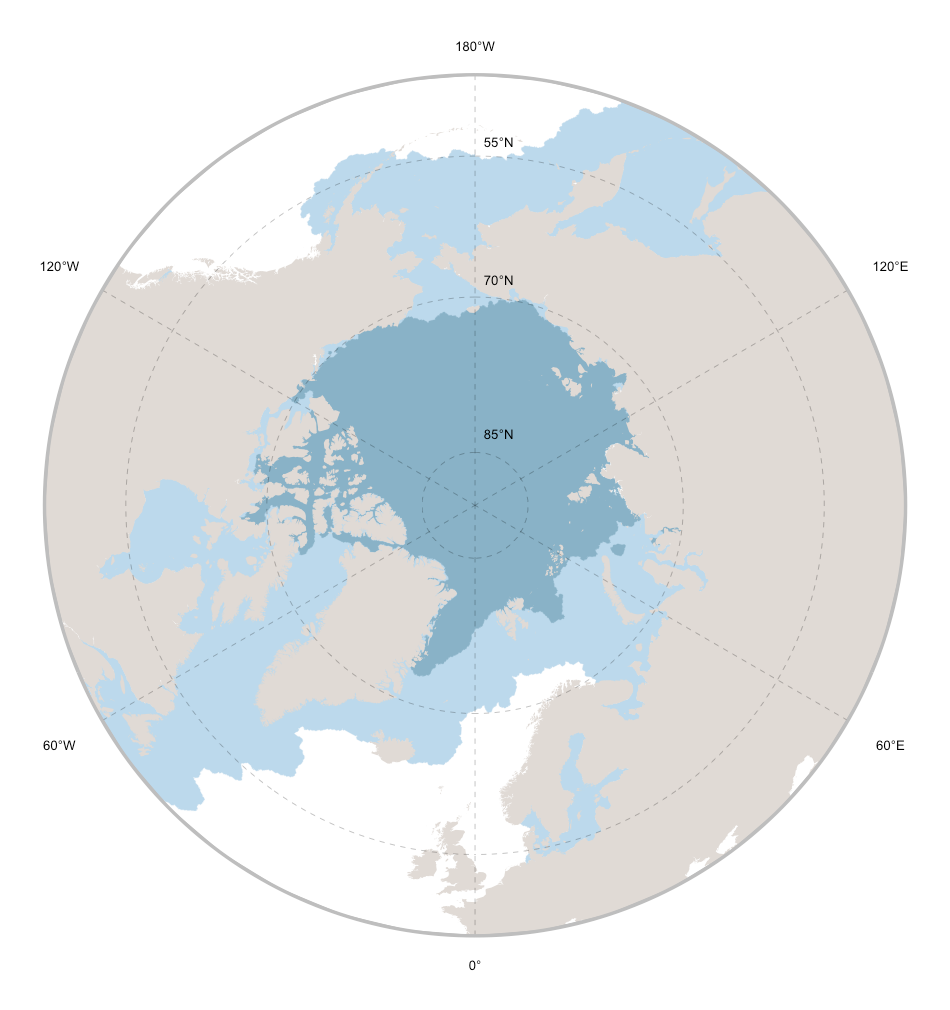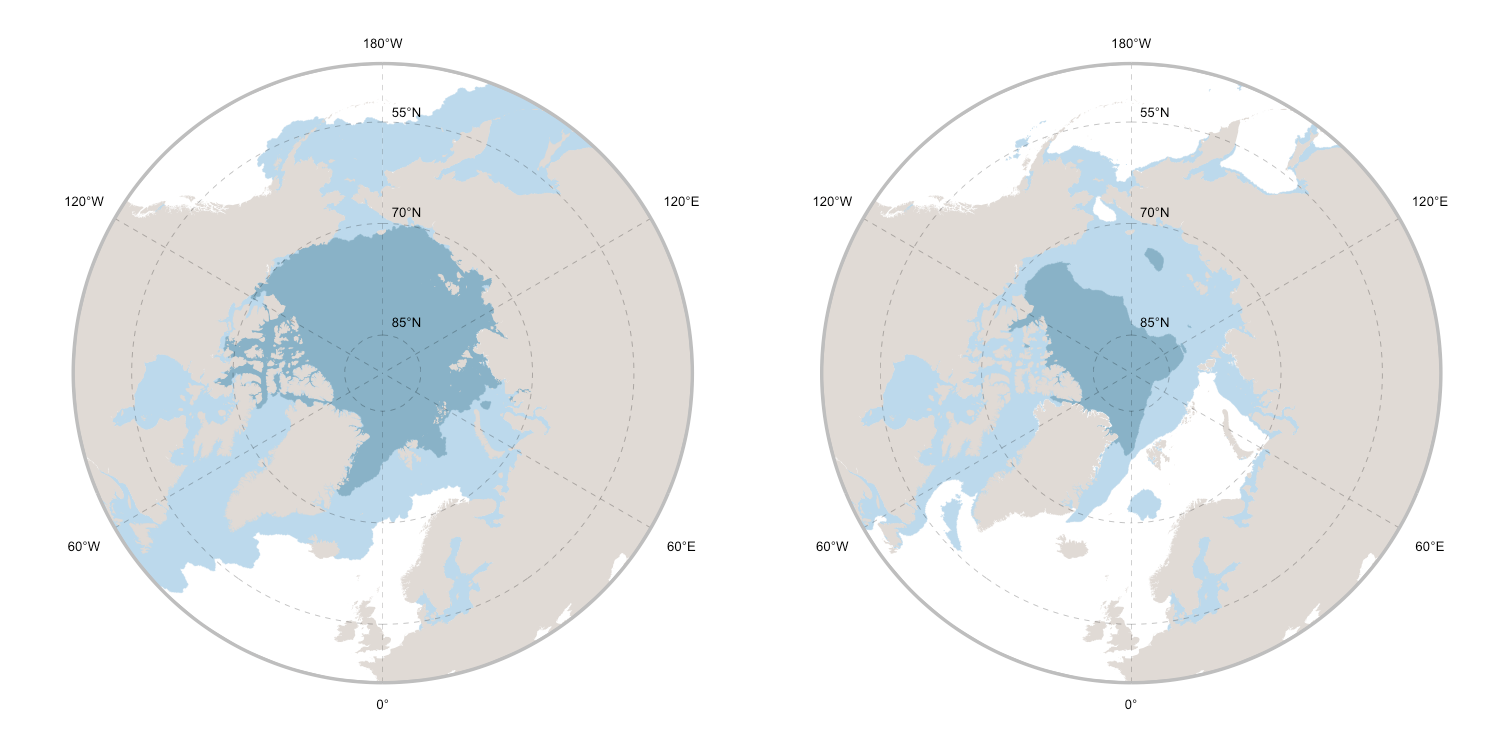Open code

### Mapping Arctic sea ice extent

Map the present-day and projected (decade 2090-2100) Arctic sea ice extent with a few lines of code.

Sea ice generates as seawater freezes, and because it is less dense than sea water, it floats throughout the surface of the ocean. Sea ice covers approximately 12% of the world’s oceans, and much of it is enclosed within the polar ice packs: the Arctic ice pack (Arctic Ocean) and the Antarctic ice pack (Southern Ocean). Polar ice packs experience significant yearly cycling linked to annual seasons, a natural process upon dependes global marine ecosystems. According to ongoing measurements, both summer ice thickness and extent are in a dramatic decline.

Here are a few lines of R code to produce a map of the Arctic sea ice extent. The code uses information extracted from Bio-ORACLE, an open source dataset of GIS layers that provides geophysical, biotic and environmental data for surface and benthic marine realms. The R package sdmpredictors facilitates data extraction from Bio-ORACLE, as well as smooth integration with the available pipelines for bioclimatic modelling.

# Prepare data
library(sdmpredictors)
library(ggplot2)
library(rnaturalearth)
library(raster)
library(sf)
library(stars)

# Define the extent of the map
cExtent <- c(-180,180,45,90)

# Load sea ice thickness raster data

# Load a polygon defining landmasses
worldMap <- ne_countries(scale = 10, returnclass = "sp")

# Transform sea ice thickness in a binomial surface depicting presence / absence of sea ice
iceMapMin[iceMapMin > 0] <- 1 ; iceMapMin[iceMapMin == 0] <- NA
iceMapMax[iceMapMax > 0] <- 1 ; iceMapMax[iceMapMax == 0] <- NA

# Transform sea ice data raster to polygon data
iceMapMin <- as_Spatial(st_as_sf(st_as_stars(iceMapMin), as_points = FALSE, merge = TRUE) )
iceMapMax <- as_Spatial(st_as_sf(st_as_stars(iceMapMax), as_points = FALSE, merge = TRUE) )

# Crop ice data to the final exent
iceMapMin <- crop(gBuffer(iceMapMin, byid=TRUE, width=0),cExtent)
iceMapMax <- crop(gBuffer(iceMapMax, byid=TRUE, width=0),cExtent)

# Crop landmasses to the final exent
worldMap <- crop(worldMap,cExtent)
worldMap <- aggregate(worldMap,dissolve=T)
worldMap <- gSimplify(worldMap, tol = 0.05, topologyPreserve = TRUE)

# polar map
x_lines <- seq(-120,180, by = 60)
iceMap <- ggplot() +
geom_polygon(data = iceMapMax, aes(x = long, y = lat, group = group), fill="#BCD9EC", colour = NA) +
geom_path(data = iceMapMax, aes(x = long, y = lat, group = group), color = "#BCD9EC", size = 0.1) +
geom_polygon(data = iceMapMin, aes( x = long, y = lat, group = group), fill="#89B2C7", colour = NA) +
geom_path(data = iceMapMin, aes(x = long, y = lat, group = group), color = "#89B2C7", size = 0.1) +
geom_polygon(data = worldMap, aes(x = long, y = lat, group = group), fill="#E0DAD5", colour = NA) +
theme(legend.position = "none") +
theme(text = element_text(family = "Helvetica", color = "#22211d")) +
theme(panel.background = element_blank(), axis.ticks=element_blank()) +
coord_map("ortho", orientation = c(90, 0, 0)) +
scale_y_continuous(breaks = seq(45, 90, by = 5), labels = NULL) +
scale_x_continuous(breaks = NULL) + xlab("") + ylab("") +
geom_text(size=3.5 , aes(x = 180, y = seq(53.3, 83.3, by = 15), hjust = -0.3, label = paste0(seq(55, 85, by = 15), "°N"))) +
geom_text(size=3.5 , aes(x = x_lines, y = (41 + c(-3,-3,0,-3,-3,0)), label = c("120°W", "60°W", "0°", "60°E", "120°E", "180°W"))) +
geom_hline(aes(yintercept = 45), size = 0.5, colour = "gray") +
geom_segment(size = 0.1,aes(y = 45, yend = 90, x = x_lines, xend = x_lines), linetype = "dashed") +
geom_segment(size = 1.2 ,aes(y = 45, yend = 45, x = -180, xend = 0), colour = "gray") +
geom_segment(size = 1.2 ,aes(y = 45, yend = 45, x = 180, xend = 0), colour = "gray") +
geom_segment(size = 0.1 ,aes(y = 55, yend = 55, x = -180, xend = 0), linetype = "dashed") +
geom_segment(size = 0.1 ,aes(y = 55, yend = 55, x = 180, xend = 0), linetype = "dashed") +
geom_segment(size = 0.1 ,aes(y = 70, yend = 70, x = -180, xend = 0), linetype = "dashed") +
geom_segment(size = 0.1 ,aes(y = 70, yend = 70, x = 180, xend = 0), linetype = "dashed") +
geom_segment(size = 0.1 ,aes(y = 85, yend = 85, x = -180, xend = 0), linetype = "dashed") +
geom_segment(size = 0.1 ,aes(y = 85, yend = 85, x = 180, xend = 0), linetype = "dashed")

iceMapWith a set of additional code lines it is possible to generate a projection of sea ice extent for the future (year 2100), here with an example under the Representative Concentration Scenario 6.0.

library(gridExtra)
grid.arrange(iceMapPresent, iceMapFuture, ncol=2)### Main reference

• Featured code